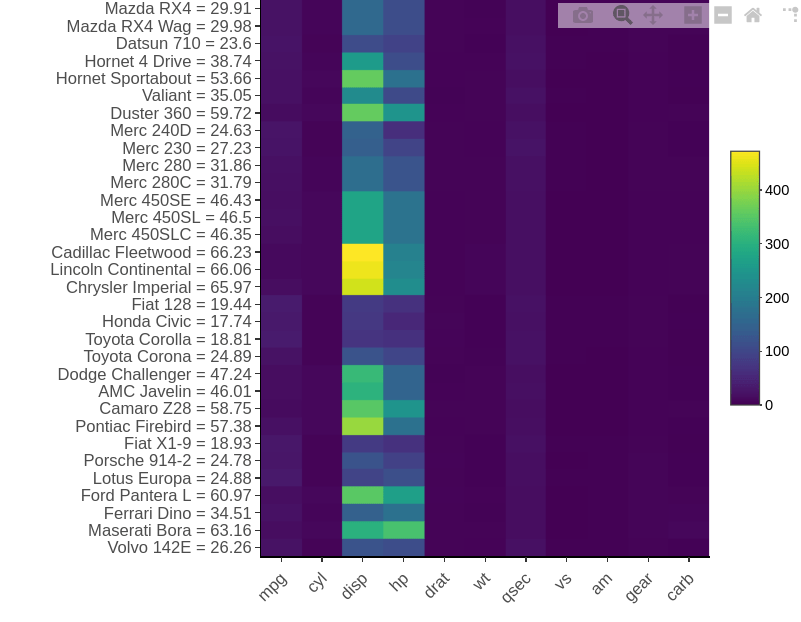# Cloud Stack Ninja

How can I add the mean values of the columns of the Heatmaply heatmap as an anotation near it?

Currently, as a not ideal approach, I run this and got the below figure:

``````x<- mtcars
mmeans <-round(rowMeans(mtcars), digits = 2 )
rownames(x) <- paste0(rownames(x)," = ", mmeans)
heatmaply(x, Rowv=F, Colv=F)
``````However, this is not ideal. I want to have the mean values as another independent column added to heat map in which I can show the values as well.

Also, I need the column label at top, which I asked here:

I am planning to use this heatmap in a shiny app.

Thanks